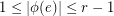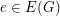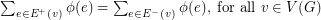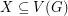flow conjectures

Circular flow numbers of $r$-graphs ★★

Author(s): Steffen

A nowhere-zero-flowonis an orientationoftogether with a functionfrom the edge set ofinto the real numbers such that, for all, and.

A-regular graphis a-graph iffor everywithodd.

Conjecture   Letbe an integer. Ifis a-graph, then.

Keywords: flow conjectures; nowhere-zero flows